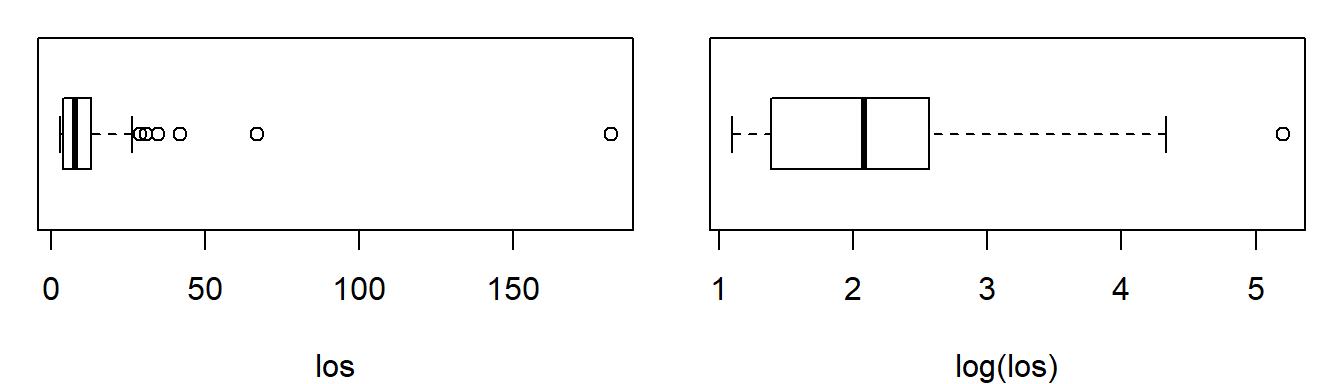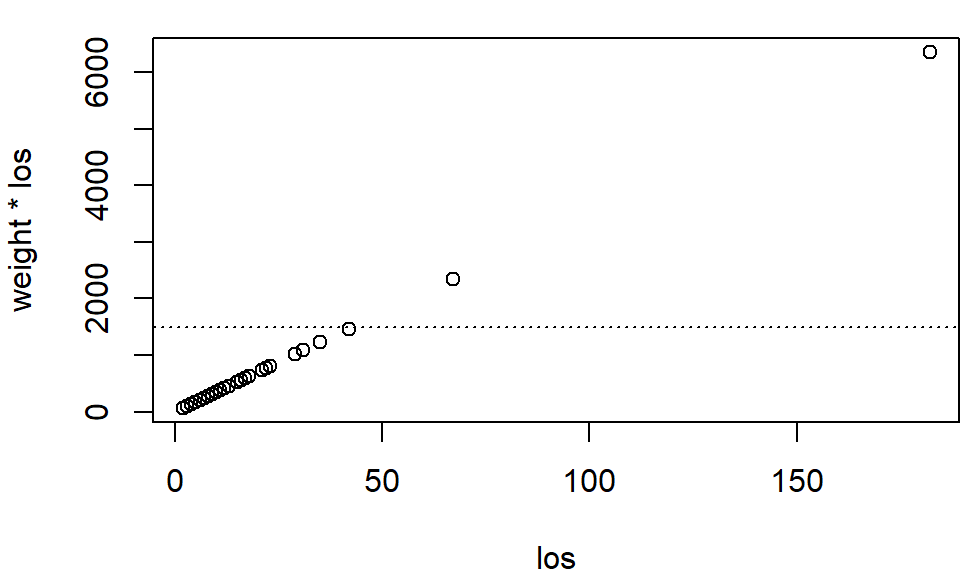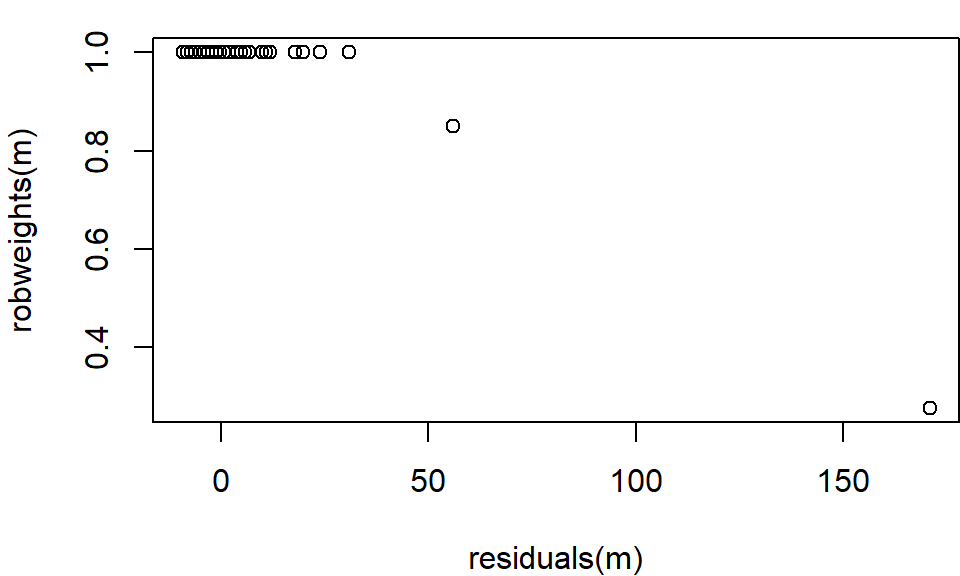## Outline

In this vignette, we discuss how to use robust estimators of location (and scale). The estimators are organized by i) estimating method, 2) population characteristic and 3) type of implemented function.

#### Estimating methods

• Trimming (Chap. 2)
• Winsorization (Chap. 3)
• Weight reduction (Chap. 4)
• M-estimation (Chap. 5)

#### Population characteristics

• mean
• total
• (standard deviation $$\rightarrow$$ see utility functions, Chap. 6)

#### Type of implementation

• bare-bone methods (only estimate)
• survey methods (estimate, standard error, variance, etc.)

Bare-bone methods are stripped-down versions of the survey methods in terms of functionality and informativeness. These functions may serve users and package developers as building blocks. In particular, bare-bone functions cannot compute variances. The survey methods are much more capable and depend—for variance estimation—on the R package survey Lumley (2021, 2010).

Good to know.

All bare-bone methods can be called with the argument info = TRUE (default: FALSE). This instructs the functions to return a list.Note 1

IMPORTANT

To avoid unnecessary repetition, we discuss the details of estimation only for the weighted trimmed mean and total. The details are the same for all estimating methods.

## 1 LOS (Length-of-stay) Hospital Data

The losdata are a simple random sample without replacement of n = 70 patients from a (fictive) hospital population of N = 2 479 patients in inpatient treatment.Note 2 First, we load the package and the data.

> library("robsurvey", quietly = TRUE)
> data("losdata")
> attach(losdata)

The first 3 rows of the data are:

> head(losdata, 3)
los   weight  fpc
1  10 34.91549 2479
2   7 34.91549 2479
3  21 34.91549 2479

where

• los length-of-stay in hospital (days)
• weight sampling weight
• fpc population size (finite population correction)

We consider estimating average length-of-stay in hospital (LOS, days).

### 1.1 Survey design object

For the survey methods (not bare-bone methods), we must load the survey package (Lumley, 2010, 2021)

> library("survey")

and specify a survey or sampling design object

> dn <- svydesign(ids = ~1, fpc = ~fpc, weights = ~weight, data = losdata)

### 1.2 Exploring the data

The distribution of variable los is skewed to the right (see boxplot), and we see a couple of rather heavy outliers. On the logarithmic scale, the distribution is slightly skewed to the right. The outliers need not be errors. Following Chambers (1986), we distinguish representative outliers from non-representative outliers.

Definition (Chambers, 1986)

• Representative outliers are extreme but correct values and are thought to represent other population units similar in value.
• A nonrepresentative outlier is an atypical or extreme observation whose value is either deemed erroneous or unique in the sense that there is no other unit like it.

The outliers visible in the boxplot refer to a few individuals who stayed for a long time in inpatient care. Moreover, we assume that these outliers represent patients in the population that are similar in value (i.e., representative outliers).The outliers tend to inflate the variance of the weighted mean. Although the outliers are not some kind of error, it is beneficiary to use estimators other than the weighted mean to estimate the population average of los. We are interested in robust estimators which—although being biased as estimators of the population mean—will often have a smaller mean square error than the weighted mean; thus, are more efficient.

## 2 Trimming

### 2.1 Bare-bone methods

The following estimation methods are available.

 weighted_mean_trimmed() weighted_total_trimmed()

In place of the weighted mean, we consider the 5% one-sided trimmed weighted population mean of los. The lower end of the distribution is not trimmed (lower bound: LB = 0). The 5% largest observations are trimmed (upper bound: UB = 0.95).

> weighted_mean_trimmed(los, weight, LB = 0, UB = 0.95)
 9.323529

We obtain an estimate of (roughly) 9.3 days. Note that the return value is a scalar.

med (upper bound: UB = 0.95).

If a bare-bone method is called with argument info = TRUE, the function returns a list, the names of which are shown below.

> m <- weighted_mean_trimmed(los, weight, LB = 0, UB = 0.95, info = TRUE)
> names(m)
 "characteristic" "estimator"      "estimate"       "variance"
 "residuals"      "model"          "design"         "call"          

### 2.2 Survey methods

The survey methods are:

 svymean_trimmed() svytotal_trimmed()

As before, we are interested in computing the 5% one-sided trimmed weighted population mean of los. In contrast to weighted_mean_trimmed(), the method svymean_trimmed() computes the standard error of the estimate using the functionality of the survey package.

> m <- svymean_trimmed(~los, dn, LB = 0, UB = 0.95)
> m
mean    SE
los 9.324 1.064

The estimated location, variance, and standard error of the estimator can be extracted from object m with the following commands.

> coef(m)
los
9.323529
> vcov(m)
Variance
los 1.131988
> SE(m)
 1.063949

The summary() method summarizes the most important facts about the estimate. The summary is particularly useful for M-estimators (see below) but less so for other estimators.

> summary(m)
Weighted trimmed estimator (0, 0.95) of the population mean

mean    SE
los 9.324 1.064

Sampling design:
Independent Sampling design
svydesign(ids = ~1, fpc = ~fpc, weights = ~weight, data = losdata)

Additional utility functions are residuals(), fitted(), and robweights(). These functions are mainly relevant for M-estimators.

## 3 Winsorization

### 3.1 Bare-bone methods

The bare-bone methods are:

 weighted_mean_winsorized() weighted_total_winsorized() weighted_mean_k_winsorized() weighted_total_k_winsorized()

We compute the 5% one-sided winsorized weighted population mean of los. The lower end of the distribution is not winsorized (lower bound: LB = 0). The 5% largest observations are winsorized (upper bound: UB = 0.95).

> weighted_mean_winsorized(los, weight, LB = 0, UB = 0.95)
 10.40845

The one-sided winsorized estimators can also be specified in absolute terms by winsorizing a fixed number $$k=1,2,\ldots$$ of observations. This estimator is called the one-sided k-winsorized mean (and total) and is computed as follows

> weighted_mean_k_winsorized(los, weight, k = 1)
 11.40845

### 3.2 Survey methods

The survey methods are:

 svymean_winsorized() svytotal_winsorized() svymean_k_winsorized() svytotal_k_winsorized()

The utility functions coef(), vcov(), SE(), summary(), residuals(), fitted(), and robweights() are available.

Good to know.

For the survey methods with postfix _winsorized, the implementation offers two variance estimation techniques.

• simple_var = FALSE: the “standard” variance estimation technique for the winsorized mean (and total). It depends on a kernel-based estimate of the density function, which is evaluated at the winsorization quantiles. Under circumstances, this estimate can be difficult to compute and/ or unreliable.
• simple_var = TRUE: a simplified variance estimation technique, which is based on the variance of the weighted trimmed mean (or total).

## 4 Weight Reduction Methods

Winsorization and trimming act directly on the values of an estimator. Other estimation methods reduce the sampling weight of potential outliers instead. A hybrid method of winsorization and weight reduction to treat influential observations has been proposed by Dalén (1987). An observation $$y_i$$ is called influential if its expanded value, $$w_iy_i$$, is exceedingly large. Let $$c>0$$ denote a winsorization or censoring cutoff value. Dalén’s estimator "Z2" and "Z3" of the population $$y$$-total are given by $$\sum_{i \in s} [w_i y_i]_{\circ}^c$$, where $$\circ$$ is a placeholder for "Z2" or "Z3" and \begin{align*} [w_i y_i]_{Z2}^c = \begin{cases} w_i y_i & \text{if} \quad w_i y_i \leq c, \\ c & \text{otherwise}, \end{cases} &\qquad \text{and} \qquad [w_i y_i]_{Z3}^c = \begin{cases} w_i y_i & \text{if} \quad w_i y_ \leq c, \\ c + (y_i - c/w_i) & \text{otherwise}. \end{cases} \end{align*}

Estimator "Z2" censors the terms $$w_iy_i$$ at $$c$$. In estimator "Z3", observations $$y_i$$ such that $$w_iy_i > c$$ contribute to the estimated total only with $$c$$ plus the excess over the cutoff, $$(w_iy_i - c)$$. Note that the excess over the threshold has a weight of 1.0 (Lee, 1995). An estimator of the population $$y$$-mean obtains by dividing the estimator of the estimated $$y$$-total by the (estimated) population size.

From a practical point of view, the choice of constant $$c$$ in Dalén’s estimators is rather tricky because we cannot only derive $$c$$ from a large order statistic, say $$y_{(k)}$$, $$k < n$$ (like for trimming). Instead, the corresponding weight $$w_{(k)}$$ needs to be taken into account.

Good to know.

It is helpful to plot $$w_iy_i$$ (weight times los) against $$y_i$$ (los). The censoring constant $$c = 1500$$ (see dotted horizontal line) is such that the two largest $$(w_iy_i)$$’s are censored to 1 500.### 3.1 Bare-bone methods

The bare-bone methods are:

 weighted_mean_dalen() weighted_total_dalen()

The estimators "Z2" and "Z3" can be specified by the argument type; by default, type = "Z2". The censoring threshold $$c$$ is implemented as argument censoring.

> weighted_mean_dalen(los, weight, censoring = 1500)
2 of 71 observations censored
 10.73129

### 4.2 Survey methods

The survey methods are:

 svymean_dalen() svytotal_dalen()

The utility functions coef(), vcov(), SE(), summary(), residuals(), fitted(), and robweights() are available.

## 5 M-Estimation

### 5.1 Bare-bone methods

The bare-bone methods are:

 weighted_mean_huber() weighted_total_huber() weighted_mean_tukey() weighted_total_tukey()

The estimators with postfix _huber and _tukey are based on, respectively, the Huber and Tukey (biweight) $$\psi$$-function.

IMPORTANT

Two types of M-estimators are available:

• type = "rwm": robust weighted mean estimator
• type = "rht": robust Horvitz-Thompson estimator of Hulliger (1995) $$\rightarrow$$ separate vignette
The robust Horvitz-Thompson estimator (type = "rht") is the method of choice for pps designs (i.e., designs without replacement where the sample inclusion probabilities are proportional to some measure of size). For equal-probability designs, the M-estimator of type = "rwm" tends to be superior.

As losdata is a simple random sample, M-estimators of type = "rht" is the method of choice. Here, we compute the Huber-type robust weighted M-estimator of the mean with robustness tuning constant $$k=8$$.

> weighted_mean_huber(los, weight, type = "rwm", k = 8)
 11.17228

Good to know.

In general, the tuning constant k must be chosen larger than (loosely speaking) “we are used to choose it” in “classical” robust statistic. More precisely, in the context of an infinite population with a standard Gaussian distribution, the constant $$k = 1.345$$ ensures that the Huber M-estimator of location achieves 95% efficiency compared with the arithmetic mean under the Gaussian model. The efficiency considerations underlying the choice of $$k = 1.345$$ do not carry over to distributions other than the Gaussian.

The M-estimators are computed by iterative methods. If the algorithm fails to converge, the functions return NA. By default, the algorithm uses a maximum of maxit = 50 iterations and a numerical tolerance criterion of tol = 1e-5 as a stopping rule. Other values of maxit and tol can be specified in the function call.

The function huber2() is an implementation of the weighted Huber proposal 2 estimator. It is only available as bare-bone method.Note 3

> huber2(los, weight, k = 8)
 13.02817

### 5.2 Survey methods

The survey methods are:

 svymean_huber() svytotal_huber() svymean_tukey() svytotal_tukey()

The Huber M-estimator of the mean (and its standard error) can be computed with

> m <- svymean_huber(~los, dn, type = "rwm", k = 8)
> m
mean    SE
los 11.17 1.328

The summary() method summarizes the most important facts about the M-estimate

> summary(m)
Huber M-estimator (type = rwm) of the population mean

mean    SE
los 11.17 1.328

Robustness:
Psi-function: with k = 8
mean of robustness weights: 0.9877

Algorithm performance:
converged in 4 iterations
with residual scale (weighted MAD): 5.93

Sampling design:
Independent Sampling design
svydesign(ids = ~1, fpc = ~fpc, weights = ~weight, data = losdata)

The estimated scale (weighted MAD) can be extracted with the scale() function. Additional utility functions are coef(), vcov(), SE(), residuals(), fitted(), and robweights(). The following figure shows a plot of the robustness weights against the residuals. We see that large residuals are downweighted.

> plot(residuals(m), robweights(m))An adaptive M-estimator of the total (or mean) is defined by letting the data chose the tuning constant $$k$$. Let $$\widehat{T}$$ denote the weighted total, and let $$\widehat{T}_k$$ be the Huber M-estimator of the weighted total with robustness tuning constant $$k$$. Under quite general regularity conditions, the estimated mean square error (MSE) of $$\widehat{T}_k$$ can be approximated by (see e.g., Gwet and Rivest, 1992; Hulliger, 1995)

$\widehat{\mathrm{mse}}\big(\widehat{T}_{k}\big) \approx \mathrm{var}\big(\widehat{T}_{k}\big) +\big(\widehat{T} - \widehat{T}_{k}\big)^2.$

The minimum estimated risk (MER) estimator (Hulliger, 1995) selects $$k$$ such that $$\widehat{\mathrm{mse}}\big(\widehat{T}_{k}\big)$$ is minimal (among all candidate estimators). Now, suppose that we have been working on the M-estimator with $$k=8$$.

> m <- svymean_huber(~los, dn, type = "rwm", k = 8)

Next, we compute the MER, starting from the current M-estimate, i.e., object m.

> mer(m)
Search interval: [1, 10]
Minimum found for k =  10
Rel. efficiency gain: 35%
mean    SE
los 11.46 1.478

Hence, the MER is 35% more efficient than the classical estimator as an estimator of the population total.

## 6 Utility Functions

### 6.1 Weighted quantile and median

The weighted quantile (and median) can be computed by

> weighted_quantile(los, weight, probs = c(0.1, 0.9))
10% 90%
3  22
> weighted_median(los, weight)
50%
8 

When all weights are equal, weighted_quantile() is equal to base::quantile() with argument type = 2.

### 6.2 Weighted MAD: median absolute deviation

The normalized weighted median absolute deviations about the weighted median can be computed with

> weighted_mad(los, weight)
 5.930408

By default, the normalization constant to make the weighted MAD an unbiased estimator of scale at the Gaussian core model is constant = 1.482602. This constant can be changed if necessary.

### 6.3 Weighted IQR: interquartile range

The normalized weighted interquartile range can be computed with

> weighted_IQR(los, weight)
 6.6717

By default, the normalization constant to make the weighted IQR an unbiased estimator of scale at the Gaussian core model is constant = 0.7413. This constant can be changed if necessary.

## Bibliographical notes

The paper of Chambers (1986) is the landmark paper about outliers in finite population sampling. Lee (1995) and Beaumont and Rivest (2009) are a good starting point to learn about robustness in finite population sampling.

Trimming and winsorization are discussed in Lee (1995) and Beaumont and Rivest (2009). The variance estimators of the weighted trimmed and winsorized estimators are straightforward adaptions of the classical estimators; see Huber and Ronchetti (2009, Chap. 3.3) or Serfling (1980, Chap. 8). A rigorous treatment in the context of finite population sampling can be found in Shao (1994).

Rao (1971) was among the first to propose weight reduction. Consider a sample of size $$n$$, and suppose that the $$i$$th observation is an outlier. He suggested to reduce the outlier’s sampling weight $$w_i$$ to one, and redistribute the weight difference $$w_i−1$$ among the remaining observations. As a result, observation $$i$$ does not represent other values like it. Dalén’s estimator offers a more general notion of weight reducution; see Dalén (1987) and also Chen et al. (2017).

In the context of finite population sampling, M-estimators were first studied by Chambers (1986). He investigated robust methods in the model- or prediction based framework of Royall and Cumberland (1981). Model-assisted estimators were introduced (for ratio estimation) by Gwet and Rivest (1992) and studied by Lee (1995), and Hulliger (1995, 1999, 2005). A recent comprehensive treatment can be found in Beaumont and Rivest (2009).

## References

BEAUMONT, J.-F. AND RIVEST, L.-P. (2009). Dealing with outliers in survey data. In: Sample Surveys: Theory, Methods and Inference ed. by Pfeffermann, D. and Rao, C. R. Volume 29A of Handbook of Statistics, Amsterdam: Elsevier, Chap. 11, 247–280. DOI: 10.1016/S0169-7161(08)00011-4

CHAMBERS, R. (1986). Outlier Robust Finite Population Estimation. Journal of the American Statistical Association 81, 1063–1069, DOI: 10.1080/01621459.1986.10478374.

DALEN, J. (1987). Practical Estimators of a Population Total Which Reduce the Impact of Large Observations. Research report, Statistics Sweden, Stockholm.

CHEN, Q., ELLIOTT, M. R., HAZIZA, D., YANG, Y., GHOSH, M., LITTLE, R. J. A., SEDRANSK, J. AND THOMPSON, M. (2017). Approaches to Improving Survey-Weighted Estimates. Statistical Science 32, 227–248, DOI: 10.1214/17-STS609.

GWET, J.-P. AND RIVEST, L.-P. (1992). Outlier Resistant Alternatives to the Ratio Estimator. Journal of the American Statistical Association 87, 1174–1182, DOI: 10.1080/01621459.1992. 10476275.

HUBER, P. J. AND RONCHETTI, E. (2009). Robust Statistics, New York: John Wiley & Sons, 2nd edition, DOI: 10.1002/9780470434697.

HULLIGER, B. (2006). Horvitz–Thompson Estimators, Robustified. In: Encyclopedia of Statistical Sciences ed. by Kotz, S. Volume 5, Hoboken (NJ): John Wiley & Sons, 2nd edition, DOI: 10.1002/0471667196.ess1066.pub2.

HULLIGER, B. (1999). Simple and robust estimators for sampling. In: Proceedings of the Survey Research Methods Section, American Statistical Association, 54–63, American Statistical Association.

HULLIGER, B. (1995). Outlier Robust Horvitz–Thompson Estimators. Survey Methodology 21, 79–87.

LEE, H. (1995). Outliers in business surveys. In: Business survey methods ed. by Cox, B. G., Binder, D. A., Chinnappa, B. N., Christianson, A., Colledge, M. J. and Kott, P. S. New York: John Wiley & Sons, Chap. 26, 503–526, DOI: 10.1002/9781118150504.ch26.

LUMLEY, T. (2021). survey: analysis of complex survey samples. R package version 4.0, URL https://CRAN.R-project.org/package=survey.

LUMLEY, T. (2010). Complex Surveys: A Guide to Analysis Using R: A Guide to Analysis Using R, Hoboken (NJ): John Wiley & Sons.

RAO, J. N. K. (1971). Some Aspects of Statistical Inference in Problems of Sampling from Finite Populations. In: Foundations of Statistical Inference ed. by Godambe, V. P. and Sprott, D. A. Toronto: Holt, Rinehart, and Winston, 171–202.

ROYALL, R. M. AND CUMBERLAND, W. G. (1981). An Empirical Study of the Ratio Estimator and Estimators of its Variance. Journal of the American Statistical Association 76, 66–82, DOI: 10.2307/2287043.

RUFFIEUX, C., PACCAUD, F. AND MARAZZI, A. (2000). Comparing rules for truncating hospital length of stay. Casemix Quarterly 2, 3–11.

SHAO, J. (1994). L-Statistics in complex survey problems. The Annals of Statistics 22, 946–967, DOI: 10.1214/aos/1176325505.

SERFLING, R. J. (1980). Approximation theorems of mathematical statistics, New York: John Wiley & Sons. DOI: 10.1002/9780470316481.

VENABLES, W. N. AND RIPLEY, B. D. (2002). Modern Applied Statistics with S, New York: Springer-Verlag, 4th edition, DOI: 10.1007/978-0-387-21706-2.